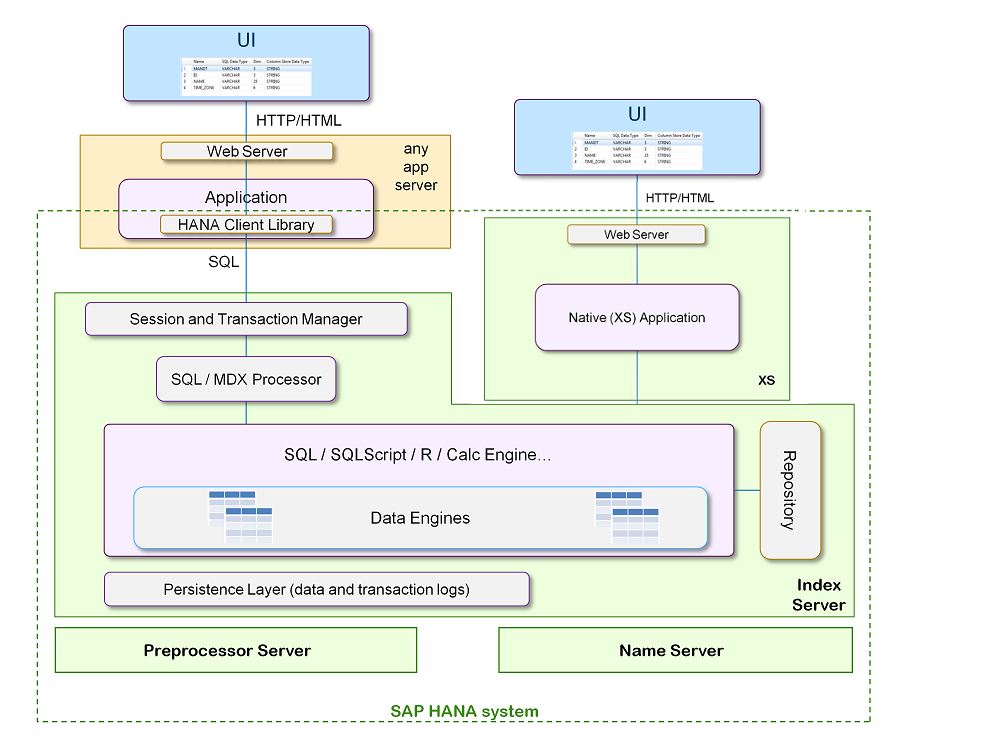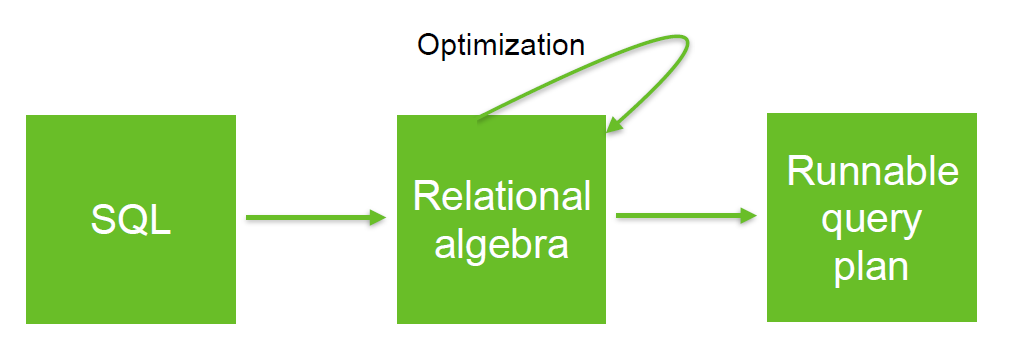# Relational Operator - Hash Joins

Hash joins are join operation used for joining large data sets.

The optimizer uses:

• the smaller of two tables or data sources
• or if the size is unknown the right-hand side

to build a hash table on the join key in memory.

It then scans the larger table, probing the hash table to find the joined rows.

This method is best used when the smaller table fits in available memory. The cost is then limited to a single read pass over the data for the two tables.

Discover MoreCollection - HashMap (Hash table)

A hash map is an implementation of a map that stores the data in buckets. The distribution of the data to a bucket is via the hash value of the key map entry (hence a HashMap). A hash map is used...HANA - Join

For most cases: HASH JOINSOracle Database - Hash joins

Hash Join The optimizer uses a hash join to join two tables if they are joined using an equijoin and if either of the following conditions are true: A large amount of data needs to be joined. ...Relational Operator - Join

join is a relational operator that pair tuples (row) of two relations (table, ...) according to a condition (predicate). where: c is a condition (ie predicate) See Type Symbol Unicode...